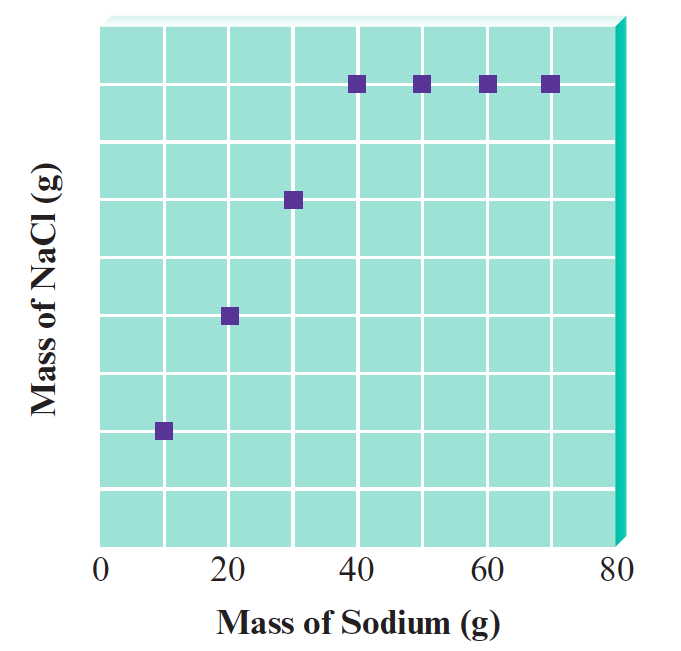# Problem: You have seven closed containers, each with equal masses of chlorine gas (Cl 2). You add 10.0 g of sodium to the first sample, 20.0 g of sodium to the second sample, and so on (adding 70.0 g of sodium to the seventh sample). Sodium and chlorine react to form sodium chloride according to the equation2 Na (s) + Cl2 (g) → 2 NaCl (s)After each reaction is complete, you collect and measure the amount of sodium chloride formed. A graph of your results is shown below.Calculate the mass of NaCl formed when 20.0 g of sodium is used.

###### FREE Expert Solution

79% (496 ratings)###### Problem Details

You have seven closed containers, each with equal masses of chlorine gas (Cl 2). You add 10.0 g of sodium to the first sample, 20.0 g of sodium to the second sample, and so on (adding 70.0 g of sodium to the seventh sample). Sodium and chlorine react to form sodium chloride according to the equation

2 Na (s) + Cl2 (g) → 2 NaCl (s)

After each reaction is complete, you collect and measure the amount of sodium chloride formed. A graph of your results is shown below.Calculate the mass of NaCl formed when 20.0 g of sodium is used.